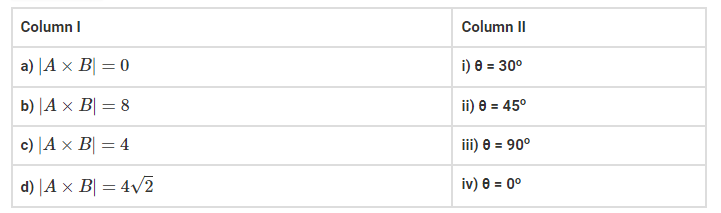# |A|=2 and |B|=4, then match the relations in`
Question:

|A|=2 and |B|=4, then match the relations in column I with the angle $\theta$ between $\mathrm{A}$ and $\mathrm{B}$ in column II.Solution:

(a) matches with (iv)

(b) matches with (iii)

(c) matches with (i)

(d) matches with (ii)